# General: Calculating Hyperfocal Distance and Depth of Field

On this page, I present how the hyperfocal distance (HFD) and depth of field (DOF) can be calculated based on formulae that I found on the DOFMaster Website. I am using Microsoft Excel for performing the actual calculations.

On other pages, I present the results my calculations of the hyperfocal distance for the Sony RX100 M1, the Ricoh GR and the Leica X Vario as well as for depth of field for the Ricoh GR and the Leica X Vario in various tabular formats.

See the notes on page A Small Glossary of Photography Terms regarding the validity of depth of field and hyperfocal distance calculations as well as of the circle of confusion, on which these calculations are based.

## Introduction

The DOFMaster Website not only offers a depth of field (DOF) calculator but also lists formulae for calculating depth of field tables on your own. Below, I list the formulae for DOF and hyperfocal distances that I use for my own calculations that are presented as tables in various places on this site.

Such calculations are, however, tedious, and error prone process. Therefore, please take the tables presented on this site and elsewhere with a grain of salt. Moreover, particularly for zoom lenses, such tables are not too useful and practical...

## The Circle of Confusion (CoC)

... might as well been regarded as a source of confusion...

The original definition of the circle of confusion was based o the resolving power of the human eye, which was assumed to be no smaller than one quarter of a millimeter in diameter on a piece of 8 x 10 paper 250 millimeters from the eye. Merklinger writes:

• The human eye is said to be capable of resolving a spot no smaller than one quarter of a millimeter in diameter on a piece of paper 250 millimeters from the eye. If this spot were on an 8 by 10 inch photograph made from a 35 mm negative, the enlargement factor used in making the print would have been about eight. Thus if spots smaller than one-quarter millimeter are unimportant in the print, then spots smaller than one-thirty-second of a millimeter in diameter are unimportant in the negative. The usual standard used in depth-of-field calculations is to permit a circle-of-confusion on the negative no larger than one-thirtieth of a millimeter in diameter. (From Merklinger)

As a result, most lenses show a DOF scale, provided they have one, that is based on a CoC of 1/30 mm (0.03 to 0.035 mm). Since this is based on a full-format film format, the CoC size has been adapted to different sensor sizes (based on the magnification factor that is much higher than 8, or on the sensor size).

## Considerations Before You Do Any Calculations

Before you do any calculations, you have to consider which focal length and aperture values are to be used in the calculations.

### Focal Length

Please note that for focal length, you have to use the exact focal length, not the equivalent length. For example, use 18 mm instead of 28 mm, which is the "equivalent" focal length for 18 mm for an APS-C camera.

### Exact Aperture Values

Aperture values are a bit more tricky. When I compared my initial results wit those of DOFMaster, there were differences for some focal lengths. I found out that there were differences, when the nominal f-numbers were rounded and did not correspond to the "exact" values, which are powers of roots of 2. DOFMaster points out: f-number is calculated by the definition N = 2i/2, where i = 1, 2, 3, ... for f/1.4, f/2, f/2.8, ... - which is true for full f-numbers only... For one-half or one-third aperture steps, it gets even more complicated:

• Full f-numbers: 2 power 1/2 = sqrt(2) = 1.414213562
• One-half f-numbers: 2 power 1/4 = fourth root of 2 = 1.189207115
• One-third f-numbers: 2 power 1/6 = sixth root of 2 = 1.122462048

Here is a table of rounded "exact" apertures in full, one-half, and one-third steps:

 Apertures Step Full One-Half One-Third 0 1.00 1.00 1.00 1 1.41 1.19 1.12 2 2.00 1.41 1.26 3 2.83 1.68 1.41 4 4.00 2.00 1.59 5 5.66 2.38 1.78 6 8.00 2.83 2.00 7 11.31 3.36 2.24 8 16.00 4.00 2.52 9 22.63 4.76 2.83 10 32.00 5.66 3.17 11 -- 6.73 3.56 12 -- 8.00 4.00 13 -- 9.51 4.49 14 -- 11.31 5.04 15 -- 13.45 5.66 16 -- 16.00 6.35 17 -- 19.03 7.13 18 -- 22.63 8.00 19 -- 26.91 8.98 20 -- 32.00 10.08 21 -- -- 11.31 22 -- -- 12.70 23 -- -- 14.25 24 -- -- 16.00 25 -- -- 17.96 26 -- -- 20.16 27 -- -- 22.63 28 -- -- 25.40 29 -- -- 28.51 30 -- -- 32.00

Table: Exact aperture values in full, one-half, and one-third steps rounded to two decimals

You get correct values for the hyperfocal distance (HFD) and depth of field (DOF) only if you use the exact f-numbers. In my tables (see elsewhere), I list both the rounded exact and the "nominal" f-numbers for your convenience.

Note: I noticed that some hyperfocal distance and depth of field calculators use "standard" aperture values. A a consequence, these deliver values that differ from my calculations, which are based on "exact" aperture values.

## Calculating the Hyperfocal Distance

According to the DOFMaster Website and Wikipedia (definition 1 of the hyperfocal distance), hyperfocal distance H is calculated using to the following formula:

• H = f*f/(N*c) + f

where f = focal length [mm], N = f-number, c = circle of confusion [mm]; H will also be in mm and has to be divided by 1,000 to deliver results in meters.

This formula can be approximated to:

• H = f*f/(N*c)

The approximation corresponds to definition 2 of the hyperfocal distance on Wikipedia (it is also mentioned on the DOFMaster Website).

See also my glossary of terms for the two different definitions of the hyperfocal distance. In the following, I use the first formula (or definition 1) for calculating the depth of field.

## Calculating the Depth of Field

The DOFMaster Website bases its formulae for the depth of field (DOF) limits on the hyperfocal distance H and calculates the near and the far DOF limits (near limit Dn, far limit Df) using the following formulae:

• Dn = s*(H-f)/(H+s-2f)
• Df = s*(H-f)/(H-s)

where H = f*f/(N*c) + f = hyperfocal distance, according to definition 1 in Wikipedia; f = focal length [mm], N = f-number, c = circle of confusion [mm], s = distance set at the lens [mm]; Dn/f values will be in mm and have to be divided by 1,000 to be in meters.

You can calculate the hyperfocal distance according to one of the two formulae above and then enter the value into the DOF formulae above. The DOF formulae based on the hyperfocal distance H are also useful for calculating the near and far limits (= the DOF) for the extreme case that distance is set to infinity. This is helpful when adopting Merklinger's approach to estimating depth-of-field in landscape photography. See below for the derivation of the results.

As with the hyperfocal distance, the circle of confusion plays (CoC) an important role in the DOF calculations. However, I transformed the formulae so that both, the formula for the near limit and the one for the far limit look, very much the same*. This similarity helped me when using Excel for calculating the DOF tables, particularly when searching for errors.

However, I prefer to arrive at formulae, in which the near and far limits depend only focal length, f-number, circle of confusion, and distance. Therefore, the hyperfocal distance needs to be substituted by its definition.

Substituting the hyperfocal distance H in the near/far limit formulae with its definition leads to:

• Dn = s*f*f/(N*c*(f*f/N*c)-f+s)
• Df = s*f*f/(N*c*(F*F+f-s)

After some transformations, I arrive as the following formulae for the near limit Dn and the far limit Df:

• Dn = s/(1+(s-f)*N*c/(f*f))
• Df = s/(1-(s-f)*N*c/(f*f))

In these formulae, the near and far limits Df/n depend only on three parameters (just like the hyperfocal distance...) plus the distance s set at the lens:

• f-number N (aperture),
• focal length f, and
• circle of confusion c (which can be regarded as an indicator of the sensor size)
• distance s set at the lens.

By the way, using the approximation for H (or definition 2), actually leads to more complex formulae and minor (or major) differences when the different calculations are compared.

Note: Like for the hyperfocal distance, exact f-numbers and real focal lengths have to be used in the calculations.

### Approximation (Wikipedia)

Wikipedia uses approximations of the formulae for DOF and hyperfocal distance to calculate Dn and Df:

• Dn = s*H/(H+s)
• Df = s*H/(H-s) for s<H

For H, it uses the approximation:

• H = f*f/(N*c)

Substituting H leads to the following approximate formulae:

• Dn = f*f*N*c*s/(f*f+N*c*s)
• Df = f*f*N*c*s/(f*f-N*c*s)

The above or these formulae lead to minor (or major) differences when the different calculations are compared.

### Special Case: Lens Focused at Infinity

The DOFMaster formulae for the near and the far depth of field limits Dn and Df are useful for inspecting a special case, namely, distance set to infinity:

• Dn = s*(H-f)/(H+s-2f) - approximation (from Merklinger): s*H/(H+s)
• Df = s*(H-f)/(H-s) - approximation (from Merklinger): s*H/(H-s)

where f = focal length [mm], H = hyperfocal distance [mm], s = distance set at the lens [mm]; Dn/f values will be in mm and have to be divided by 1,000 to be in meters. Now we let s go towards infinity:

• Dn = s*H/(H+s-2f) - s*f/(H+s-2f) = H/(H/s+1-2f/s) - f/(H/s+1-2f/s) => s=>inf => Dn = H/(0+1-0) - f/(0+1-0) = H - f = H
• Df = s*H/(H-s) - s*f/(H-s) = H/(H/s-1) - f/(H/s-1) => s=>inf =>Df = H/(0-1) - f/(0-1) = -H + f = -H

The approximations lead to just Dn = H, Df = -H. A negative HFD means a distance beyond infinity, that is, sharpness is at infinity.

Thus, as Merklinger does (and Boone as well), we can state that, when we focus any lens at infinity, the near limit (based on the CoC criterion!) is the hyperfocal distance and the far limit is infinity.

If you do not believe my calculations, you can simply look at the photos below: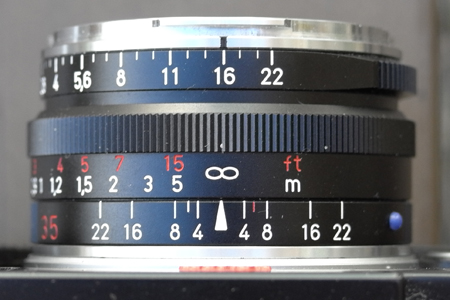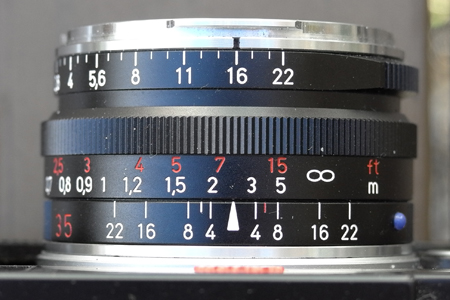Set the distance to infinity and read the near distance or hyperfocal distance from the "near" mark - here f/16 Move the infinity mark to the "far" marker for the chosen f-number - here f/16 - to set the hyperfocal distance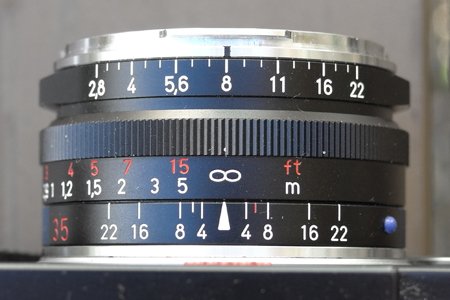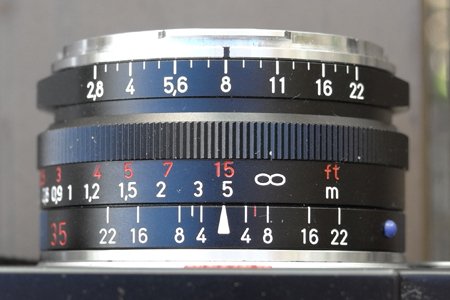Set the distance to infinity and read the near distance or hyperfocal distance from the "near" mark - here f/8 Move the infinity mark to the "far" marker for the chosen f-number - here f/8 - to set the hyperfocal distance

Photos: When distance is set to infinity, the "near limit" is just the hyperfocal distance, as the photos in the right column prove...

## Why Do I Get/Find Different Values from the Ones Published on this Site?

You may have found a Website that offers a DOF/hyper focal distance calculator, or you bought an app that performs these and other calculations. Then you stumble across this Website to find out that the DOF and hyper focal distance values published here differ from the ones that you calculate or read. This is, of course, confusing, and you may rightfully ask who is wrong. In the following, I would like to propose a few answers to this puzzle:

• Hyperfocal distance can be calculated according to two formulae/definitions (see Willie). These differ in only one term - the focal length. Thus, the difference between both variants is small, but it exists (it's just the focal length).
• When using formulae for DOF, you can calculate the hyperfocal distance first and then enter this value into the respective formulae for the near and far limit (DOF). Depending on which of the two formulae you use, whether you round the value before entering it into the formulae for the near and far limits, you can arrive at slightly different values.
• Wikipedia lists simplified, approximate formulae for calculating DOF. If you use these you will also get slightly different DOF values…
• DOFMaster points out that you not only have to use the actual focal length but also the exact f-values in the calculations, which is a power of 2 (the exponent depends on the size of the steps). I found a number of sites/apps that use the "nominal" f-numbers (those that are written on the lenses) for the calculations (you may nevertheless specify the nominal values…). For f-numbers that are not plain powers of 2 (these are f/2, f/4, f/8, f/16, f/32), you get values that differ from the DOFMaster values. Therefore, when I find that a calculator more less replicated the results of the DOFMaster formulae, I check this not only for an aperture value of f/4, but also for f/2.8 (which is 2*sqrt(2) = 2.828…).

All these differences are minor, but they exist and they can puzzle you. They can also add up…

In my opinion, every application or Website that wants to be trusted should disclose the formulae according to which it calculates its values. This allows its users/visitors to check the validity of the results and to understand why there are differences. Actually, after fiddling around a lot with hyperfocal distance and DOF data, I feel a bit as in the saying "trust only those statistical data that you faked yourself" (or so…). After looking at so many DOF/hyperfocal distance apps/sites, I tend to trust only my own calculations — and those that they are based on, that is, the DOFMaster Website formulae and calculations/calculator.

### ExifTool

ExifTool lists "Composite" tags at the end of the Exif data that it supplies (the same does the long-time Mac imaging tool GraphicConverter), among others, the hyperfocal distance (HFD). I therefore assumed that such a thing as a "composite Exif tag" does exist and that some cameras write the hyperfocal distance into the Exif data. I was, however, wrong, as I found out on the ExifTool Website. Author Phil Harvey writes:

• The values of the composite tags are Derived From the values of other tags. These are convenience tags which are calculated after all other information is extracted.

For calculating the hyperfocal distance, Harvey uses the FocalLength, Aperture, and CircleOfConfusion tags in the Exif data. This may explain, why I detected differences between the value in the composite HFD tag and my own calculations based on the DOFMaster formulae. I assume, that Harvey does not use the exact aperture value, which is based on powers of two, but directly the one given in the Exif data. Since the deviations can be in either direction, the calculated values may be sometimes too short and sometimes too wide (they should not be taken too strictly anyway...). I checked my assumption but did not arrive at a conclusion yet...

## Conclusions

The formulae for the near and far distance that I have derived may look even more complex to you than the original ones, but they offer the advantage that they differ only in one sign, which is very helpful when using Excel. The formulae based on the approximations presented in Wikipedia do not look much simpler either, after the hyperfocal distance was substituted by its definition...

Note that you can also calculate the hyperfocal distance first (either according to definition 1 or 2) and then enter this value into the DOF formulae (the ones given here from DOFMaster or the approximations listed in Wikipedia). I prepared a HFD/DOF calculator ins Excel using all these approaches in order to compare the approximations with the formulae that I use. Overall, the differences are minor...

## Alternative: Merklinger's Approach

In his article Hyperfocal distances and Merklinger's method in landscape photography, Kevin Boone discusses hyperfocal distance versus Harold M. Merklinger's approach to estimating depth-of-field in landscape photography. He also discusses simpler approaches, such as using f/8 and setting distance to infinity.

I prepared a page, entitled Merklinger's Approach to Estimating Depth of Field, that covers Merklinger's approach in more detail. In addition, I calculated tables for using a special and simple case of the method for most of my cameras. You will find these pages in the respective sections.

## References

### Merklinger

 gerd (at) waloszek (dot) de About me made by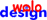on a mac!
 27.05.2016